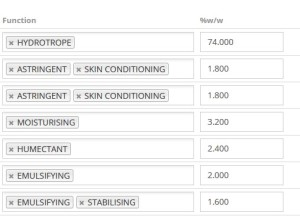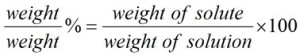FREE ONLINE SOFTWARE DEMO AND TRIAL

# Using %w/w percentage weight concentrations in cosmetic formulas

Homecosmetri product blogUsing %w/w percentage weight concentrations in cosmetic formulas

To use cosmetri it is important that you understand the principle of entering %w/w amounts in your formula’s composition. This is the most universally accepted method for formulation of cosmetic products and the method that is used in cosmetri.In percent solutions, the weight of a solute is expressed as a percentage of the total solution in weight. By solute we mean, a raw material that is used in your formula, whereas solution refers to the total resultant mixture of your product.

### The %w/w formula is expressed as follows:Note that ‘weight’ refers to mass (i.e., as measured on scales).

If a raw material in your formula is a liquid and measured by volume, you must know the mass of this, which requires a density value.

### %w/w concentrations - example:

An oil in your formula has a density of 0.9 g/ml. If the total mass of your solution is 100 g, you first need to know the mass of the oil. If you were making 100 g of your product and add 50 ml of the oil during manufacture, this will be 50 x 0.9 g/ml = 45 g. Therefore, in 100g of your product’s solution, 45 g of this will be your oil, meaning that it has a %w/w of 45.000.

Using %w/w is the simplest form of entering a formula’s composition because by using weights consistently throughout the formulation, the total mass of the solution is always equal to the sum of the mass of each of the solutes. In other words, if you add 45.000 g of a solute dissolved in 55.000 g of solvent, you will have a resulting solute concentration of 45.000 % and an exact mass of 100.000 g.

Entering percentage ranges for raw material compositions

### Related Posts## New Product Manager Version (v.3.9.3)## Cosmetics GMP Software now Available## New Formulation and Costing Tools now Available (v.3.9.1)